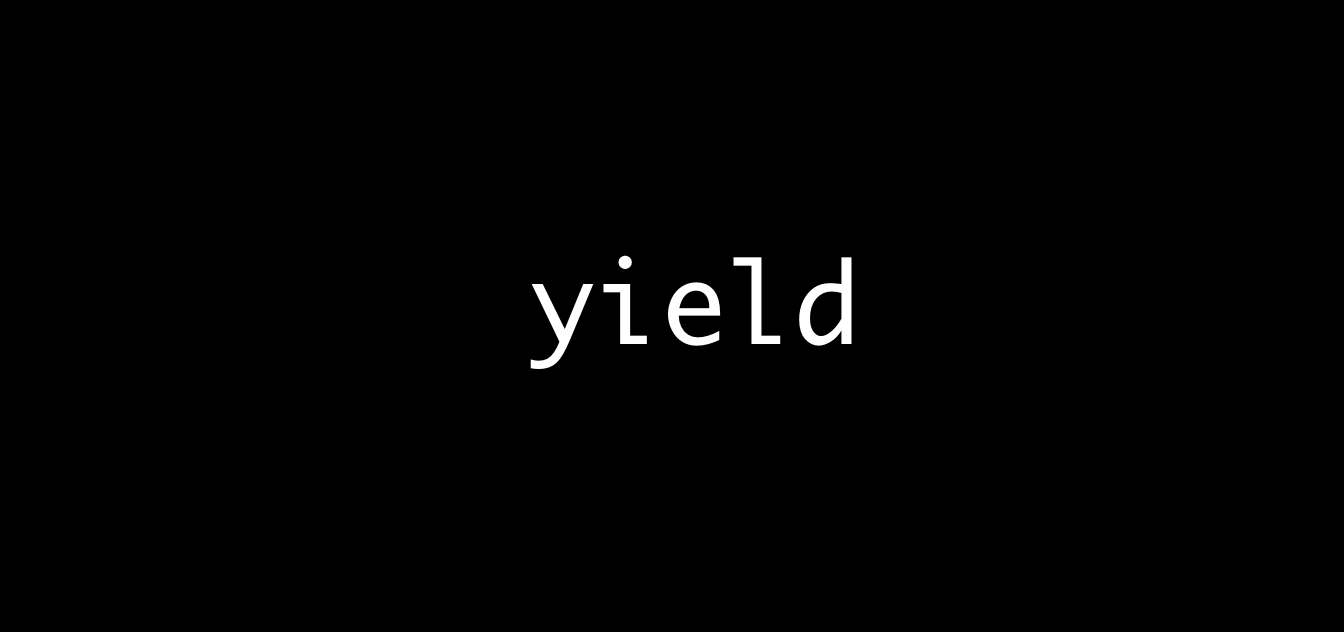## 什么是 Coroutine？

Coroutine 可以被翻译为协程，它与 Subroutine（子程序）有什么区别呢？其实我们绝大多数接触到的函数也好，方法也好，都是 Subroutine 的一种，它的特点就是，子程序从函数入口开始，一直到 return 语句结束，也就是说，一旦子程序返回了，它这一阶段的所有使命就完成了。

## 它与线程有什么关系？

Now in (Language)JS

## 初见 Coroutine

``````function fibonacii() {
let i = 1;
let j = 1;
for (let k = 0; k < 10; k++) {
console.log(i);
console.log(j);
i += j;
j += i;
}
}
``````

``````function *fibonaciiUsingYield() {
let i = 1;
let j = 1;
while (true) {
yield i;
yield j;
i += j;
j += i;
}
}
``````

``````const gen = fibonaciiUsingYield();
gen.next();
``````

next 函数返回一个对象，包含了每次迭代的结果和一个可否继续迭代的 flag。

## 手动写一个 Coroutine

C 语言中很好实现跳转，goto 语句完事。在 JavaScript 中我们可以通过 switch case 的方式达到相同的目的。

JavaScript 中就很好实现了，由于有闭包，我们完全可以将执行体放到一个闭包里，然后在闭包外声明所有所需的局部变量和状态信息，让闭包自动捕获它们。所以一个简单的实现方式就出来了：

``````function genFibonaciiCoroutine() {
let i = 1;
let j = 1;
let state = 1;
return function () {
let retVal;
switch (state) {
case 0:
i += j;
j += i;
state = 1;
case 1:
retVal = i;
state = 2;
break;
case 2:
retVal = j;
state = 0;
break;
}
return retVal;
}
}
``````